## Norm

Given a-D Vectora Vector Normis a Nonnegative number satisfying
1.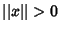when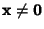and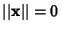Iff,

2.for any Scalar,

3.The most common norm is the vector L2-Norm, defined byGiven a Square Matrix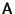, a Matrix Normis a Nonnegative number associated withhaving the properties

1.whenand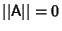Iff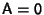,

2.for any Scalar,

3.,

4.See also Bombieri Norm, Compatible, Euclidean Norm, Hilbert-Schmidt Norm, Induced Norm, L1-Norm, L2-Norm, L(infinity)-Norm, Matrix Norm, Maximum Absolute Column Sum Norm, Maximum Absolute Row Sum Norm, Natural Norm, Normalized Vector, Normed Space, Parallelogram Law, Polynomial Norm, Spectral Norm, Subordinate Norm, Vector Norm

References

Gradshteyn, I. S. and Ryzhik, I. M. Tables of Integrals, Series, and Products, 5th ed. San Diego, CA: Academic Press, pp. 1114-1125, 1979.